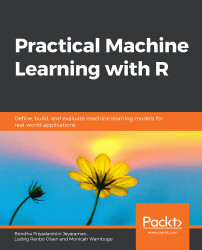•#### Practical Machine Learning with R#### Overview of this book

With huge amounts of data being generated every moment, businesses need applications that apply complex mathematical calculations to data repeatedly and at speed. With machine learning techniques and R, you can easily develop these kinds of applications in an efficient way. Practical Machine Learning with R begins by helping you grasp the basics of machine learning methods, while also highlighting how and why they work. You will understand how to get these algorithms to work in practice, rather than focusing on mathematical derivations. As you progress from one chapter to another, you will gain hands-on experience of building a machine learning solution in R. Next, using R packages such as rpart, random forest, and multiple imputation by chained equations (MICE), you will learn to implement algorithms including neural net classifier, decision trees, and linear and non-linear regression. As you progress through the book, you’ll delve into various machine learning techniques for both supervised and unsupervised learning approaches. In addition to this, you’ll gain insights into partitioning the datasets and mechanisms to evaluate the results from each model and be able to compare them. By the end of this book, you will have gained expertise in solving your business problems, starting by forming a good problem statement, selecting the most appropriate model to solve your problem, and then ensuring that you do not overtrain it.Free Chapter
An Introduction to Machine LearningData Cleaning and Pre-processingFeature EngineeringIntroduction to neuralnet and Evaluation MethodsLinear and Logistic Regression ModelsUnsupervised LearningAppendix## Regression and Classification with Decision Trees

We can solve regression and classification tasks with a multitude of machine learning algorithms. In the previous chapter, we used neural networks, and, in this chapter, we have used linear and logistic regression. In the next chapter, we will learn about decision trees and random forests, which can also be used for these tasks. While linear and logistic regression models are usually easier to interpret, random forests can sometimes be better at making predictions. In this section, we will apply random forests to our dataset and compare the results to our linear and logistic regression models.

As we have seen in Chapter 1, An Introduction to Machine Learning, a decision tree is basically a set of if/else statements arranged as an upside-down tree, where the leaf nodes contain the possible predictions. For a specific observation, we could end up with the following paths down a tree:

• If a home is larger than 1,500 sqft and it has more than...